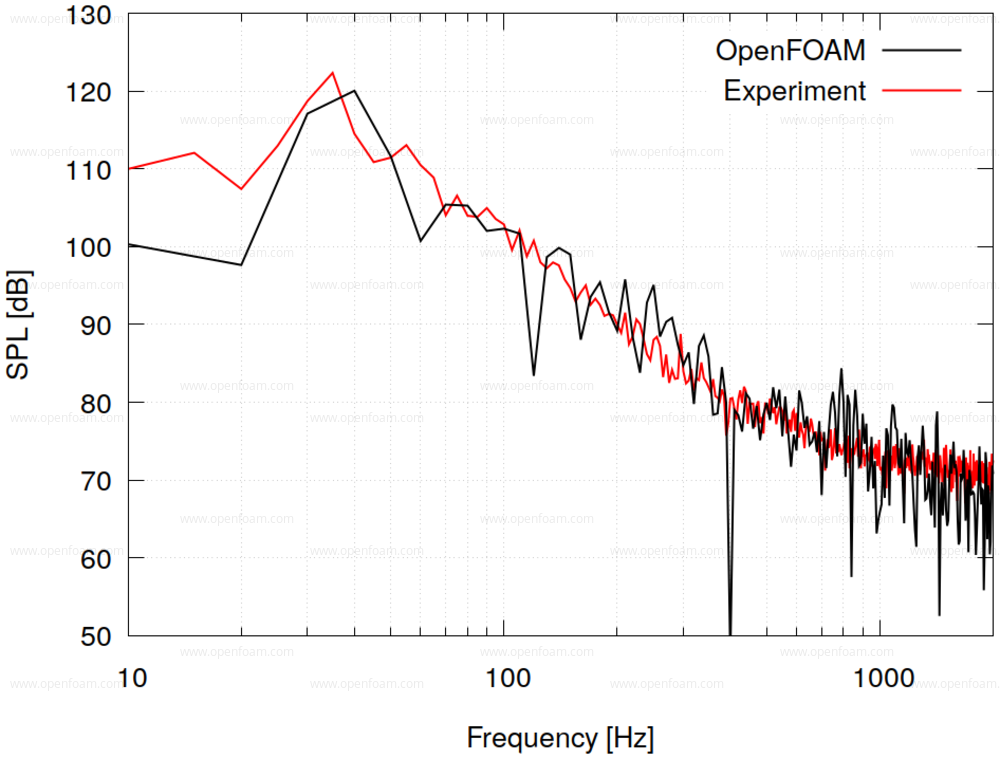## Point-based noise🔗

Example input:

noiseModel      pointNoise;

pointNoiseCoeffs
{
// Common entries (see above)

// Input files list
files       (<file-path-1> <file-path-2> <file-path-N>);
}


The point noise model can only be run in serial mode.

Pressure data is read in CSV data format

• point data generation: probes

Output:

• Text files
• Prms_f.dat : frequency vs RMS pressure [Pa]
• PSD_dB_Hz_f.dat : frequency vs Power Spectral Density [dB/Hz]
• PSD_f.dat : frequency vs Power Spectral Density [Pa$$^2$$/Hz]
• SPL_dB_f.dat : frequency vs Sound Pressure Level [dB]
• SPL13_dB_fm.dat : centre frequency vs 1/3 octave Sound Pressure Level [dB]

The example below shows a SPL comparison between OpenFOAM and experimental data for the signal recorded from a vehicle side window:Source code

API: